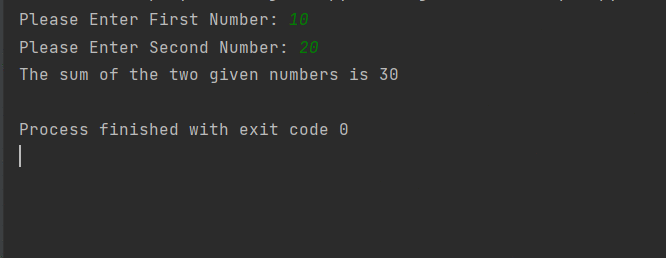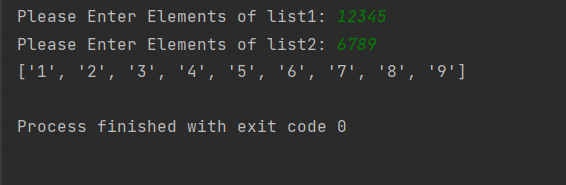Open in App
Not now

# Python input() Function

• Difficulty Level : Medium
• Last Updated : 12 Dec, 2022

Python input() function is used to take user input. By default, it returns the user input in form of a string.

## input() Function

Syntax:

`input(prompt)`

prompt [optional]: any string value to display as input message

Ex: input(“What is your name? “)

Returns: Return a string value as input by the user.

```By default input() function helps in taking user input as string.
If any user wants to take input as int or float, we just need to typecast it.```

Refer to all datatypes and examples from here.

## Python3

 `# Taking input as string` `color ``=` `input``(``"What color is rose?: "``)` `print``(color)`   `# Taking input as int` `# Typecasting to int` `n ``=` `int``(``input``(``"How many roses?: "``))` `print``(n)`   `# Taking input as float` `# Typecasting to float` `price ``=` `float``(``input``(``"Price of each rose?: "``))` `print``(price)`

Output:

```What color is rose?: red
red
How many roses?: 10
10
Price of each rose?: 15.50
15.5```

### Example 1: Taking the Name and Age of the user as input and printing it

By default, input returns a string. So the name and age will be stored as strings.

## Python

 `# Taking name of the user as input` `# and storing it name variable` `name ``=` `input``(``"Please Enter Your Name: "``)`   `# taking age of the user as input and` `# storing in into variable age` `age ``=` `input``(``"Please Enter Your Age: "``)`   `print``(``"Name & Age: "``, name, age)`

Output:

```Please Enter Your Name: Rohit
Name & Age:  Rohit 16 ```

### Example 2: Taking two integers from users and adding them.

In this example, we will be looking at how to take integer input from users. To take integer input we will be using int() along with Python input()

## Python

 `# Taking number 1 from user as int` `num1 ``=` `int``(``input``(``"Please Enter First Number: "``))`   `# Taking number 2 from user as int` `num2 ``=` `int``(``input``(``"Please Enter Second Number: "``))`   `# adding num1 and num2 and storing them in` `# variable addition` `addition ``=` `num1 ``+` `num2`   `# printing` `print``(``"The sum of the two given numbers is {} "``.``format``(addition))`

Output:Similarly, we can use float() to take two float numbers. Let’s see one more example of how to take lists as input

### Example 3: Taking Two lists as input and appending them

Taking user input as a string and splitting on each character using list() to convert into list of characters.

## Python

 `# Taking list1 input from user as list` `list1 ``=` `list``(``input``(``"Please Enter Elements of list1: "``))`   `# Taking list2 input from user as list` `list2 ``=` `list``(``input``(``"Please Enter Elements of list2: "``))`     `# appending list2 into list1 using .append function` `for` `i ``in` `list2:` `    ``list1.append(i)`   `# printing list1` `print``(list1)`

Output:My Personal Notes arrow_drop_up
Related Articles